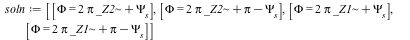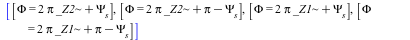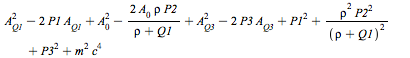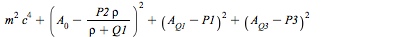## 1546 Reputation

12 years, 43 days

## A problem with a result from solve...

Maple

I have the following equation:

Diff(W(t), t) = -q*V*(sin(Phi)-sin(Psi[s]))/(2*h*Pi);

I solve it for rhs() = 0:

soln := solve([rhs((2)) = 0, Phi < 2*Pi], [Phi], allsolutions = true,explicit);

This works and I get this result:Now I want to get the first zeros, which occur for _Z1 and _Z2 equal to 0. So I substitute:

subs(_Z2=0,(3));

and getIn other words, the substitution did not work.

The original problem is embedded in a larger sheet created with Maple 15 and there it does work. It fails on Maple 2015.2. I then pulled out the relevant pieces to make this example demonstrating the problem (see the attached sheet, which has some of my other (unsuccessful) attempts to diagnose what is going on). It seems like the created variables _Z1 and _Z2 are somehow not recognized at all.

The only way I can get the _Z2 terms out is to substitute 2=0. This is really too icky to seriously consider, though.

Anyone has seen this before?

FWIW: Maple 2015.2 on Mac OS X 10.10.5.

Thanks,

Mac Dude

Assumptions_test.mw

## How to unhide input?...

Maple

I have run into a problem with a file I am preparing for a class. The file is in Document mode with one large section and a number of subsections. Input is 2-d input.

The first subsection behaves normally, input is there, output is there and so on.

The second section does not display the input. The input is still there, somewhere, as the sheet recalculates properly and I can also see there is input when looking at the file with an editor. But no show in Maple.

The third subsection does not show input either.

The fourth subsection is normal again and shows input.

Each of these sections is several screens long. The file actually is an older one where I am adding subsections. The first one (that works normal) is a new one. The original file (before I edited it to add a subsection) works fine still.

So I assume I inadvertently did something that causes this, even though the old subsections are not being edited. I do load Physics[Vectors] but cannot believe that does it. The original file also loads it.

The file was created in Maple 15 and that is where I edited this one. I opened it in Maple 2015 on a different computer, but the behaviour is identical.

Again, the input is there somewhere; the sheet recalculates corrrectly, but I cannot edit the input in the sections that do not show it (I guess that is not a surprise, but it excludes things like a white font color on a white background as there is nothing to select).

Does anyone have a hint how to unhide the input? I tried many things (menu, contextual menu, prefs,...) but no help. It is actually kind-of annoying.

FWIW, this is on Mac OS X, 10.10.5 Yosemite for Maple 2015.1. And 10.5.8 for Maple 15.

Thanks,

M.D.

## Simplification question...

Maple

I have a polynomial expression that I would like to cast into a specific form. The expression isand I know that it can be simplified into a form involving squares of (A[Qi]-Pi). It is trivial to do this on paper; how can I convince Maple to do this.

The solution I came up with was to use mtaylor and expand about the forms I know to be there:

mtaylor((3),[A[Q1]=P1,A=P2*rho/(rho+Q1),A[Q3]=P3],6);which is what I want (close to, anyway). Now, I consider this to be a bit of a dirty trick that works here as the expression is simple and no higher-order terms are present so in fact the solution is exact. But, are there methods along simplify and friends that can do this? I have not been successfull with those...

This is a part of a much longer worksheet and part of a lecture, so I need Maple to be able to do this. The mtaylor trick works, but I would not want to miss an obvious approach that may work where mtaylor would get confused.

Thanks,

M.D.

test.mw

## How to define a linear function?...

Maple

I ran into a problem with define that I traced to the 'linear' option in the define statement, and I would like to know whether this is my lack of understanding define, or a bug.

I want to define a function called avg(parm) (which I never will need to use explicitly but  I need to be able to manipulate). This function has the property of being linear, and terms not depending on x can be pulled out. Also, avg(parm)=parm unless parm depends on x. (Yes, it stands for a kind of averaging, but that is not relevant here).

So I programmed it in this way:

define(avg,'linear','conditional'(avg(a::algebraic) = a, _type(a, freeof(x))),\
'conditional'(avg(a::(nonunit(algebraic))*b::(nonunit(algebraic))) = a*avg(b), _type(a, freeof(x))));

For the heck of it I could not get it to work. Basically, none of the equations in the definition were ever used, except that constant numeric factors were pulled out, as they should.

Eventually I found that the 'linear' option was the culprit. Once I got rid of that, the definition of avg did what I wanted it to do (like avg(a*x) => a*avg(x); avg(a) => a). The reason a constant numeric factor was pulled out before is because the 'linear' option does that; it never gets to my definitions (one can see that when printing avg).

Am I missing something fundamental here, or is define not working right?

For the record, I did this in Maple 15 on a PPC Mac. I don't have my Maple 2015 handy to test on that version.

Any insights?

Mac Dude

## 2-D Input vs 1-D Input...

Maple

I have run into a "funny" feature of 2-D input: It seems to convert something like k/2 into this k*`^`(2,-1). While this would often not be an issue (it is correct after all), it becomes a problem when used in an argument list to a procedure. It becomes even more of a problem when, by chance, I have overloaded `^` to act on specific types that I have defined.

Let me try to explain briefly. I have a package called "Lattice" that does whatever it does (not of relevance here). I am writing a little manual for this package, for which I use 2-D input so I can write it in Maple and have the examples right in it and "live".

Here is what happens:

QFh:=Quad(0,kf/2) # Define an element for Lattice

Error, invalid input: Lattice:-`^` expects its 1st argument, element, to be of type Element, but received 2

Copy-pasting kf/2 into a 1-d worksheet, I get

So it uses Lattice[`^`] which actually appears to bypass the overload I have in the Lattice package. `^` is defined like this in Lattice:

`^`:=proc(element::Element,n::algebraic) option overload; # Element is a defined type in Lattice
...
end proc;

How can I possibly rewrite `^` to fall-back to Maple's ^ operator when called as Lattice[`^`] ?? I know there is a function overload() but have no experience with it. Would it even help?

Or am I missing something completely here? I do not use 2-D input for my usual work, but in this case I want and need to use it. The reason for its bizarre rewrite of "/2" is beyond me. Note that I can replace /2 by *0.5; but that causes problems later on for algebraic work as 1/2 is not 0.5 in Maple. I tried *1/2 but that has the same problem.

Has anyone a clean solution for this? I assume this effect is not limited to my own package but would affect others as well.

M.D.

PS: I ran into this using Maple 15 but I doubt it is specific to this particular version.

﻿